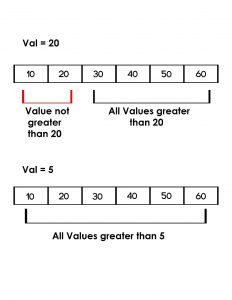# Python | Check if all the values in a list that are greater than a given value

• Last Updated : 21 Nov, 2018

Given a list, print all the values in a list that are greater than the given valueExamples:

```Input : list = [10, 20, 30, 40, 50]
given value = 20
Output : No

Input : list = [10, 20, 30, 40, 50]
given value = 5
Output : Yes
```

Attention geek! Strengthen your foundations with the Python Programming Foundation Course and learn the basics.

To begin with, your interview preparations Enhance your Data Structures concepts with the Python DS Course. And to begin with your Machine Learning Journey, join the Machine Learning - Basic Level Course

## Recommended: Please try your approach on {IDE} first, before moving on to the solution.

Method 1: Traversal of list

By traversing in the list, we can compare every element and check if all the elements in the given list are greater than the given value or not.

 `# python program to check if all ``# values in the list are greater ``# than val using traversal`` ` `def` `check(list1, val):``     ` `    ``# traverse in the list``    ``for` `x ``in` `list1:`` ` `        ``# compare with all the values``        ``# with val``        ``if` `val>``=` `x:``            ``return` `False` `    ``return` `True``     ` `     ` `# driver code ``list1 ``=``[``10``, ``20``, ``30``, ``40``, ``50``, ``60``]``val ``=` `5``if``(check(list1, val)):``    ``print``"Yes"``else``:``    ``print``"No"`` ` `val ``=` `20` `if``(check(list1, val)):``    ``print``"Yes"``else``:``    ``print``"No"`

Output:

```Yes
No
```

Method 2: Using all() function:

Using all() function we can check if all values are greater than any given value in a single line. It returns true if the given condition inside the all() function is true for all values, else it returns false.

 `# python program to check if all ``# values in the list are greater``# than val using all() function`` ` `def` `check(list1, val):``    ``return``(``all``(x > val ``for` `x ``in` `list1))``     ` `     ` `# driver code ``list1 ``=``[``10``, ``20``, ``30``, ``40``, ``50``, ``60``]``val ``=` `5``if``(check(list1, val)):``    ``print``"Yes"``else``:``    ``print``"No"`` ` `val ``=` `20` `if` `(check(list1, val)):``    ``print``"Yes"``else``:``    ``print``"No"`

Output:

```Yes
No
```

My Personal Notes arrow_drop_up